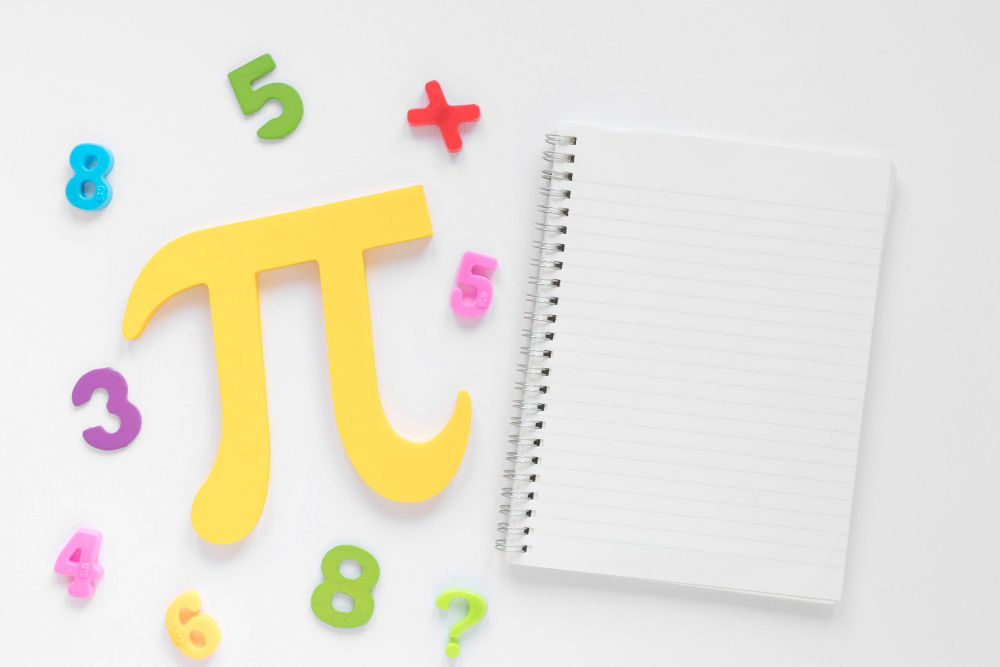As kids learn primary school math, they’re likely to hear of the famous “Pi number”.

We’ll give you a spoiler alert: while it may sound similar, a Pi number does not refer to actual pies.

Pi is a universal math concept known across the world. It was first discovered over 4,000 years ago and it’s still a major constant in the math world today!

It’s so famous that it even has a national holiday dedicated to it – Pi Day!

In this article, we’ll discuss the meaning of the Pi number and how kids can encounter it while learning math.

After reading this, children will be more prepared to face the famous number in the future!### What is the Pi number?

Pi, symbolized by the Greek letter π, represents the ratio of the circumference of a circle to its diameter. The universal number is 3.14.

Pi has been considered as a special number by mathematicians, scientists, and enthusiasts for centuries – and with good reason.

Because, if you draw a circle and divide the circumference by its diameter, you will always get an approximate equal result of 3.14.

Another special fact about Pi is that it is literally endless.

“Is Pi a rational number?” is a common question in the math community. In other words, can it be converted into fractions of whole, natural numbers?

The Pi full number does not stop itself at a value of 3.14. The number continues to go on without ever ending.

Mathematicians have calculated the digits of Pi into more than a million digits, and it continues to baffle and intrigue them.

To avoid confusing everyone, the universal value is approximately 3.14. But, its actual irrational numbers are far longer than that.

### Example questions

You might wonder how children can encounter the famous number in their studies.

As mentioned earlier, Pi is the ratio of a circle’s circumference divided by its diameter. In other words, the formula looks like this:

π = circumference / diameter

But, it is also useful when you want to find a circle’s circumference or diameter.

Here are the formulas to make that happen:

Circumference = π x diameter

Diameter = π x circumference

For instance, here is a sample question that uses the Pi number.

“Andy jogged around a circle which has a diameter of 100 m. How far did he jog?”

Here are the steps to solve it:

• Circumference = 3.14 x 100
• Circumference = approx. 314 meters

The number can also be used when children are learning about circles and other math shapes, starting from Primary 6.

### Prepare your child to learn Pi with Practicle!

Learning the Pi number early on allows your child to be more prepared to face it in the future.

If they are eager to start learning, however, you can let them try out Practicle’s Singapore math learning game!

By transforming difficult math problems into fun games, Practicle allows children to enjoy learning math on their own using their devices.

The game not only follows the syllabus from Singapore’s MInistry of Education, but it has also been endorsed by the Education Alliance of Finland!

You can witness a glimpse of the game with our 7-day free trial today.